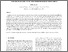# Thermoelasticity with thermomechanical constraints

Scott, N. (2001) Thermoelasticity with thermomechanical constraints. International Journal of Non-Linear Mechanics, 58 (3). pp. 549-564. ISSN 1878-5638Preview PDF (paper1.pdf) - Published Version Download (181kB) | Preview

## Abstract

Equations are derived governing the behaviour of small disturbances superimposed on an underlying equilibrium configuration of a thermoelastic body. The body may be materially inhomogeneous, non-homogeneously prestrained and be subjected to a non-uniform temperature resulting in non-constant-coefficient partial differential equations. These equations are generalized to the case where thermomechanical constraints are present, both deformation-temperature and deformation-entropy constraints. It is known that the first of these types of constraints leads to material instabilities and the second does not. By examining nearly constrained materials, and taking an appropriate limit, we find that the instabilities associated with deformation-temperature constraints arise because the heat capacity at constant deformation becomes negative whilst deformation-entropy constraints are stable because the same heat capacity remains positive, though tending to zero in the limit of the constraint holding exactly. The ten important moduli of thermoelasticity are examined in the limit of each constraint holding exactly. It is found, for example, that for each type of constraint the heat capacity at constant stress remains positive and bounded away from zero. Results on wave propagation are also presented.

Item Type: Article Faculty of Science > School of Mathematics Vishal Gautam 18 Mar 2011 14:23 15 Dec 2022 01:51 https://ueaeprints.uea.ac.uk/id/eprint/20814 10.1016/S0020-7462(00)00084-6View Item